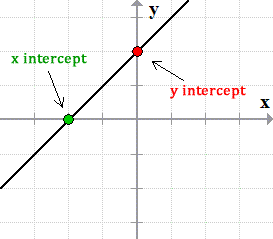# MS Math – Week of Oct 25-286th Grade Math – Mrs. Evans

This week we will finish Chapter 2 with a test on Tuesday.   This test focuses, largely, on multiplying and dividing fractions and mixed numbers.  For students who need additional help or practice with these skills, please contact Mrs. Evan s or go to IXL.COM – sections H and I.

Beginning on Wednesday we will be learning about how we can represent real world quantities as negative integers and rational numbers.  It is useful to be able to represent every situation as either positive or negative in order to think of them in a mathematical way.  We will spend time making sure that we can order positive and negative rational numbers properly before we put them into our tool box for all our future use!

Math Grade Math – Mrs. Evans and Mrs. VonFeldt

This week we will finish Module 3, which is also the conclusion of Unit 1.  Upon completion of this unit, students will have the ability to use rational numbers (fractions, decimals and integers) that are both negative and positive, in all the basic operations.  They will have practiced, in lesson 3.6, this week, to apply a strategy that will help them in solving real world problems that deal with both decimals and fractions. The Unit 1 Test will be this Friday. For any students who feel the need for additional practice on the skills we have learned in Unit 1, please go to IXL.COM – section H.

8th Grade Math – Mrs. Ernest

Students have been learning how to graph from an equation or a data table. This week we will be expanding our knowledge of linear equations, functions in general and the coordinate plane.

https://www.cuemath.com/algebra/linear-equations/

Algebra – Mrs. VonFeldt

We are continuing to explore the question: How can we use linear relationships to solve problems? A graph is one representation of a function. This week, students will learn to graph linear equations using two different strategies: 1) make a table and 2) graph using intercepts. If the x and y-intercepts are known, or can be easily found, then a straight line going through those points makes a line:We will also begin exploring slope. What is slope and how can you calculate it between two points? This will lead us into the slope-intercept form next week.© 2023 Assumption Catholic School | 2116 Cornwall Ave, Bellingham, WA 98225
Phone: 360.733.6133 | Fax: 360.647.4372
Email: theoffice@school.assumption.org
Dashboard | Web design and development by Olywebdev.com